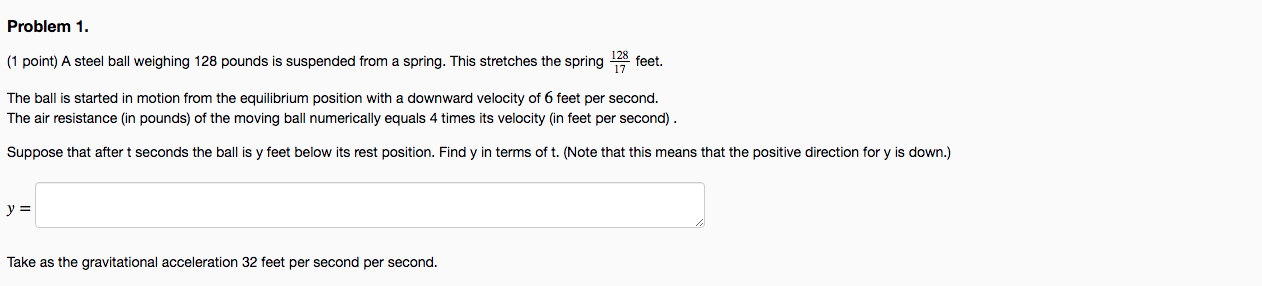I AM STRUGGLING HEAVILY ON THIS PROBLEM PLEASE HELP ME WITH THE CORRECT ANSWER AND NEAT WORK PLEASE PLEASE AND THANK YOU

Problem 1. (1 point) A steel ball weighing 128 pounds is suspended from a spring. This stretches the spring 178 feet. The ball is started in motion from the equilibrium position with a downward velocity of 6 feet per second. The air resistance (in pounds) of the moving ball numerically equals times its velocity (in feet per second) Suppose that after t seconds the ball is y feet below its rest position. Find y in terms of t. (Note that this means that the positive direction for y is down.) y = Take as the gravitational acceleration 32 feet per second per second.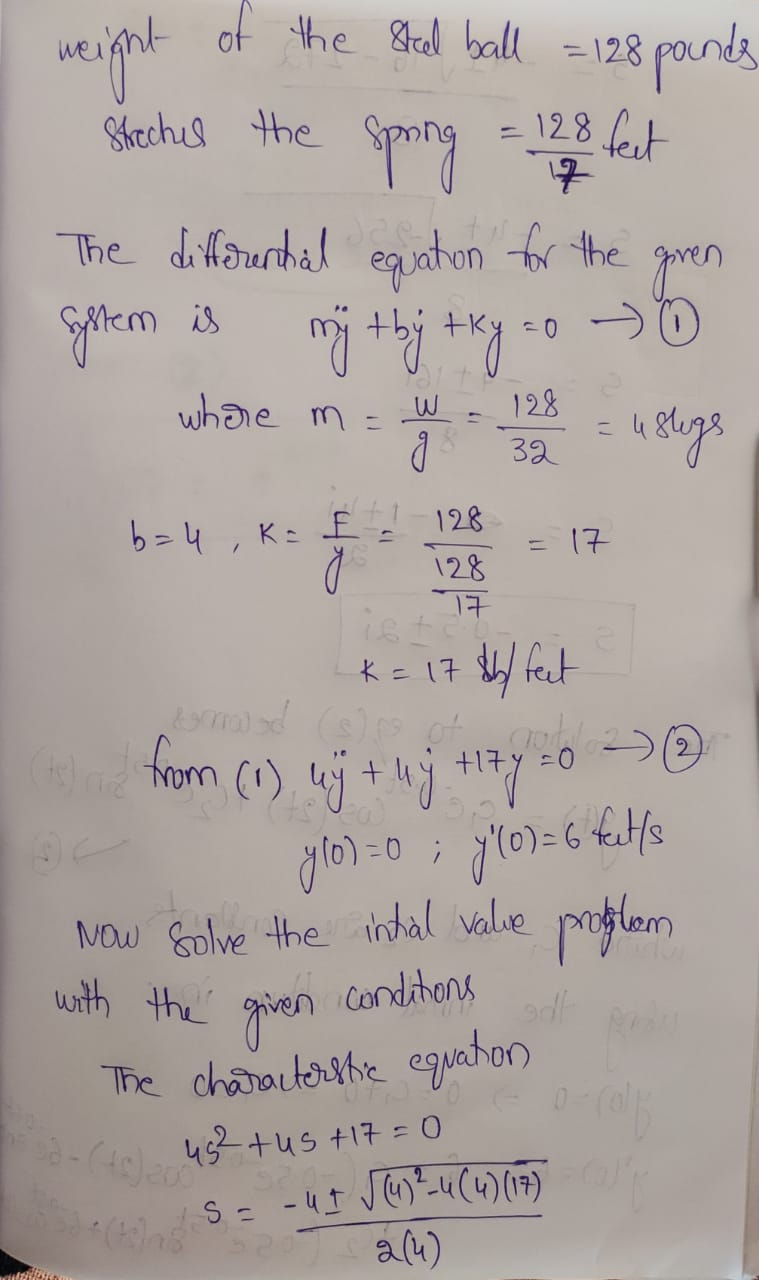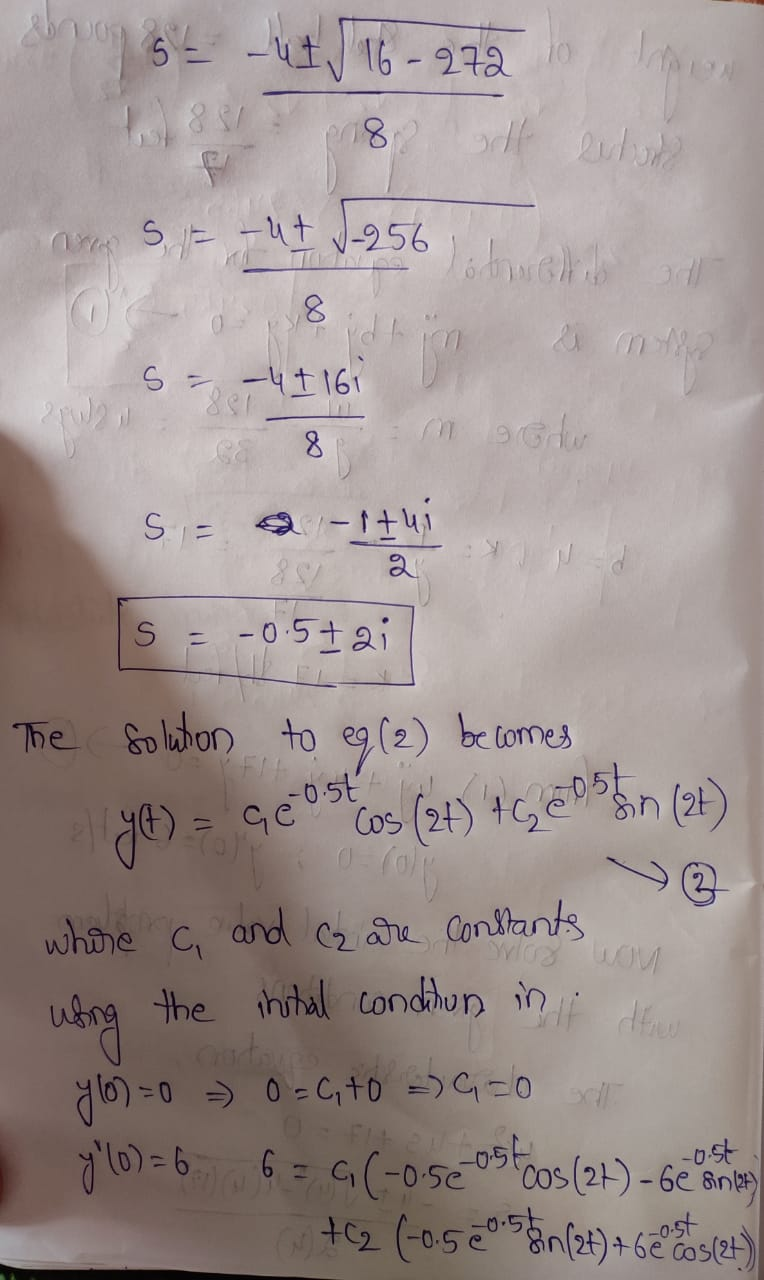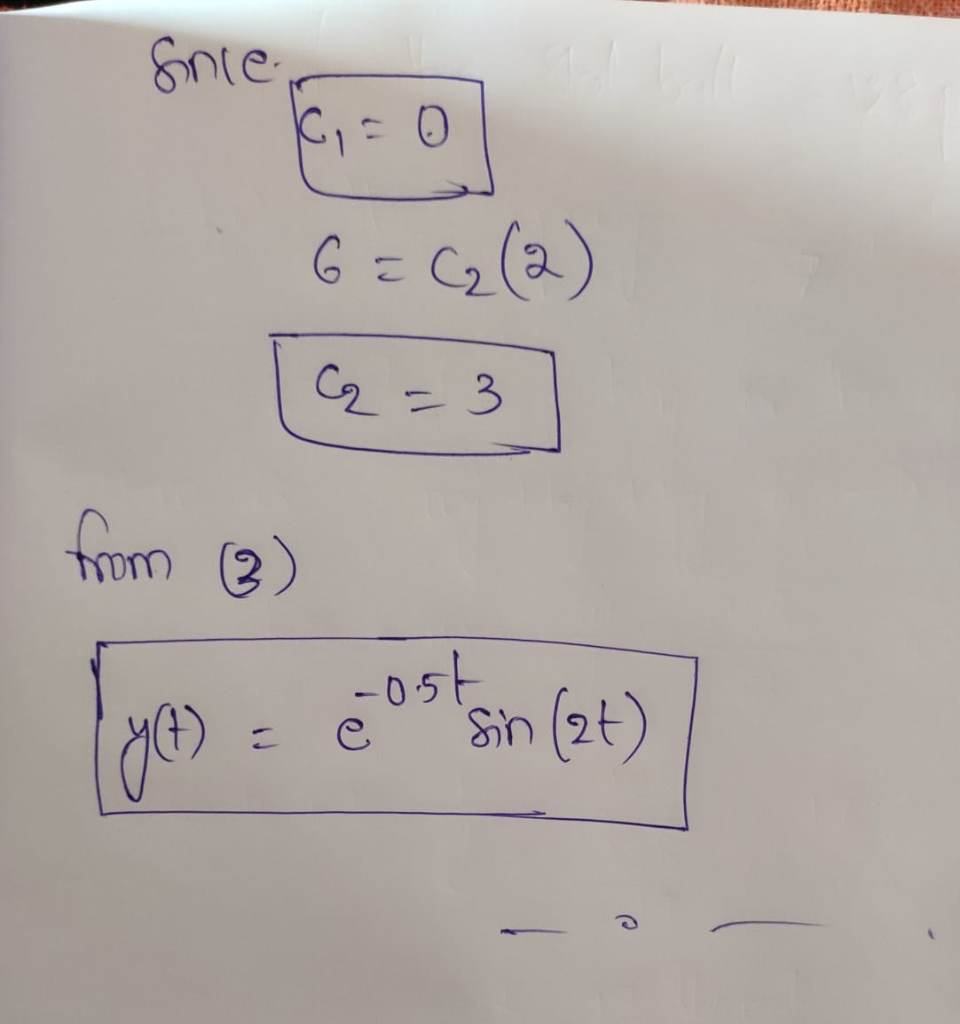THANK YOU STAY SAFE

PLEASE UPVOTE IF U LIKE THE WORK

Similar Homework Help Questions
• ### I AM REALLY STRUGGLING ON THIS PROBLEM PLEASE HELP ME CORRECT AND NEAT WORK IS MUCH...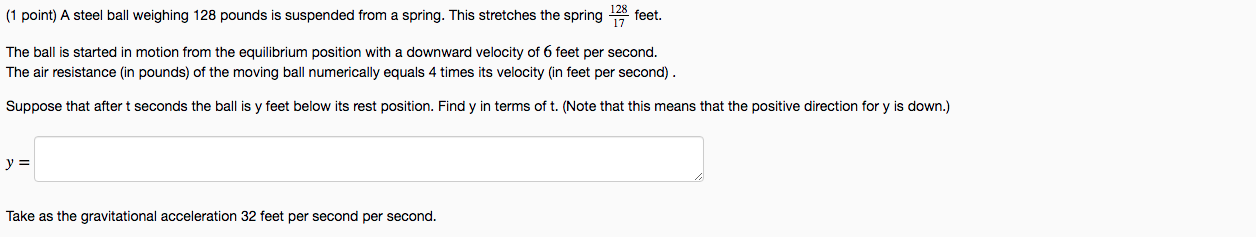I AM REALLY STRUGGLING ON THIS PROBLEM PLEASE HELP ME CORRECT AND NEAT WORK IS MUCH APPRECIATED THANKS (1 point) A steel ball weighing 128 pounds is suspended from a spring. This stretches the spring 13 feet. The ball is started in motion from the equilibrium position with a downward velocity of 6 feet per second. The air resistance (in pounds) of the moving ball numerically equals 4 times its velocity (in feet per second). Suppose that after t seconds...

• ### STRUGGLING WITH THESE TWO SETS OF PROBLEMS ID APPRECIATE THE HELP AND ALL CORRECT ANSWERS PLEASE...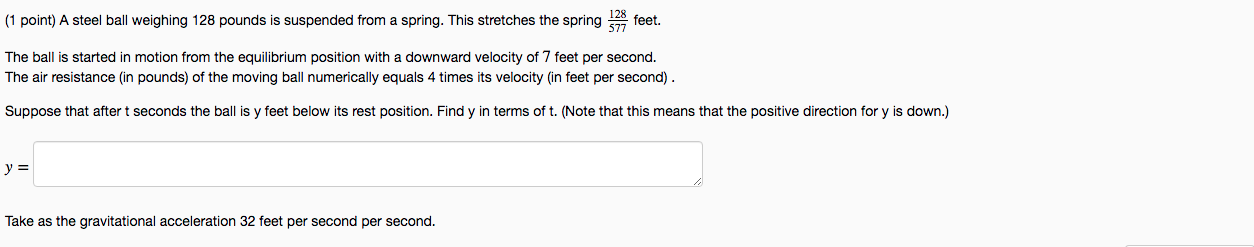STRUGGLING WITH THESE TWO SETS OF PROBLEMS ID APPRECIATE THE HELP AND ALL CORRECT ANSWERS PLEASE (1 point) A steel ball weighing 128 pounds is suspended from a spring. This stretches the spring 528 feet. The ball is started in motion from the equilibrium position with a downward velocity of 7 feet per second. The air resistance (in pounds) of the moving ball numerically equals 4 times its velocity (in feet per second). Suppose that after t seconds the ball...

• ### Problem 1. 128 325 feet. (1 point) A steel ball weighing 128 pounds is suspended from...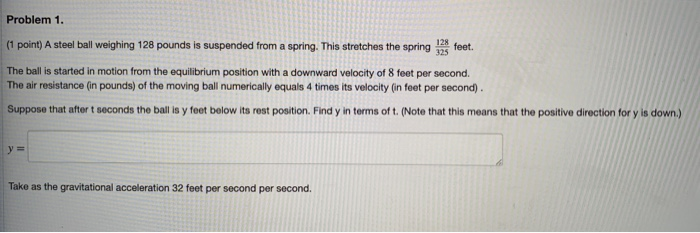Problem 1. 128 325 feet. (1 point) A steel ball weighing 128 pounds is suspended from a spring. This stretches the spring The ball is started in motion from the equilibrium position with a downward velocity of 8 feet per second. The air resistance (in pounds) of the moving ball numerically equals 4 times its velocity (in feet per second) Suppose that after t seconds the ball is y feet below its rost position. Find y in terms of t....

• ### 145 (1 point) A steel ball weighing 128 pounds is suspended from a spring. This stretches...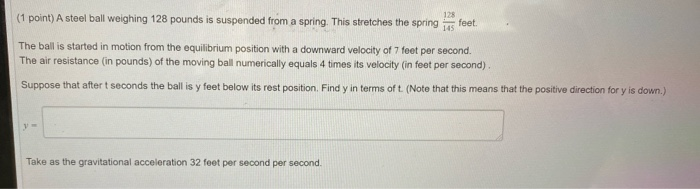145 (1 point) A steel ball weighing 128 pounds is suspended from a spring. This stretches the spring 128 feet The ball is started in motion from the equilibrium position with a downward velocity of 7 feet per second. The air resistance (in pounds) of the moving ball numerically equals 4 times its velocity (in feet per second). Suppose that after t seconds the ball is y feet below its rest position. Find y in terms of t. (Note that...

• ### (1 point) A steel ball weighing 128 pounds is suspended from a spring. This stretches the...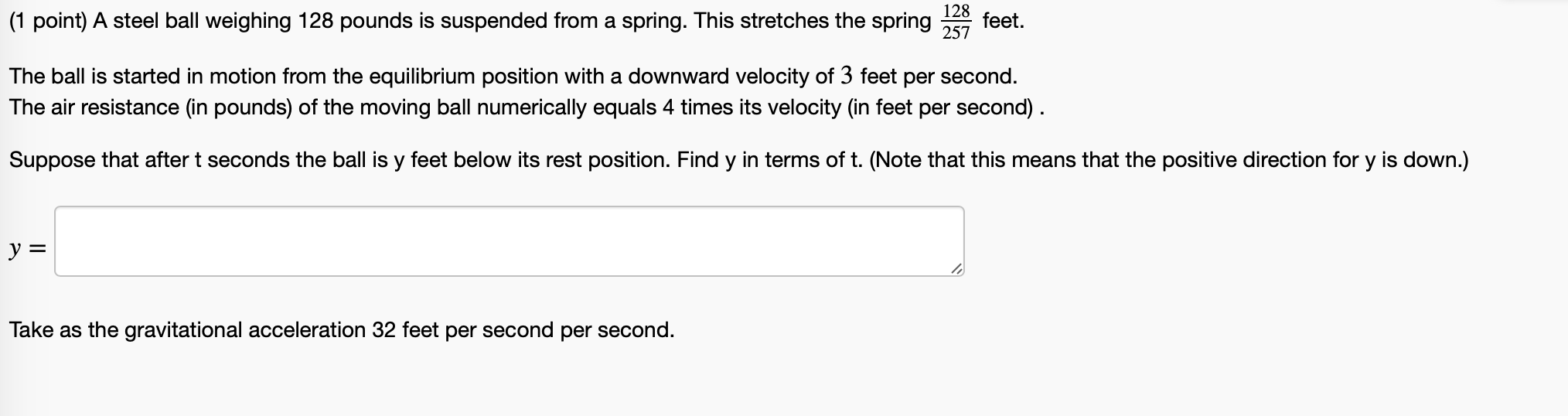(1 point) A steel ball weighing 128 pounds is suspended from a spring. This stretches the spring 128 257 feet. The ball is started in motion from the equilibrium position with a downward velocity of 3 feet per second. The air resistance (in pounds) of the moving ball numerically equals 4 times its velocity (in feet per second). Suppose that after t seconds the ball is y feet below its rest position. Find y in terms of t. (Note that...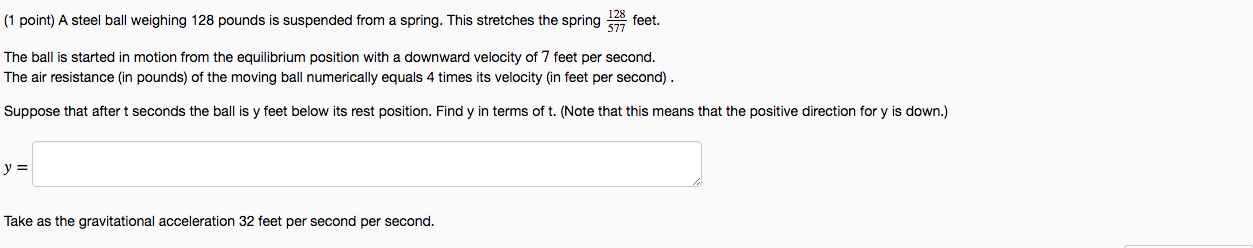PLEASE ANSWER BOTH PROBLEM SETS PLEASE ANSWER BOTH (1 point) A steel ball weighing 128 pounds is suspended from a spring. This stretches the spring 528 feet. The ball is started in motion from the equilibrium position with a downward velocity of 7 feet per second. The air resistance (in pounds) of the moving ball numerically equals 4 times its velocity (in feet per second). Suppose that after t seconds the ball is y feet below its rest position. Find...

• ### (1 point) A steel ball weighing 128 pounds is suspended from a spring. This stretches the...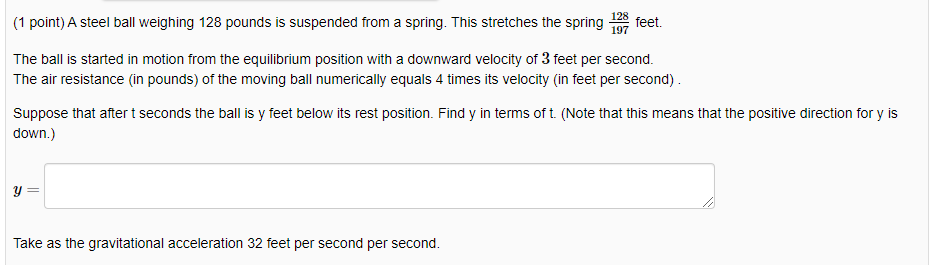(1 point) A steel ball weighing 128 pounds is suspended from a spring. This stretches the spring feet. The ball is started in motion from the equilibrium position with a downward velocity of 3 feet per second. The air resistance (in pounds) of the moving ball numerically equals 4 times its velocity (in feet per second). Suppose that after t seconds the ball is y feet below its rest position. Find y in terms of t. (Note that this means...

• ### (1 point) A steel ball weighing 128 pounds is suspended from a spring This stretches the...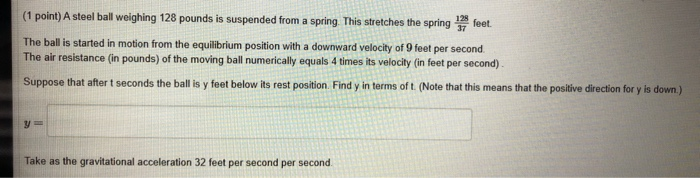(1 point) A steel ball weighing 128 pounds is suspended from a spring This stretches the spring 13 feet The ball is started in motion from the equilibrium position with a downward velocity of 9 feet per second The air resistance (in pounds) of the moving ball numerically equals 4 times its velocity (in feet per second) Suppose that after t seconds the ball is y feet below its rest position Find y in terms of t. (Note that this...

• ### Defined that natural length is unknown, can we assume its zero? do we need natural length?...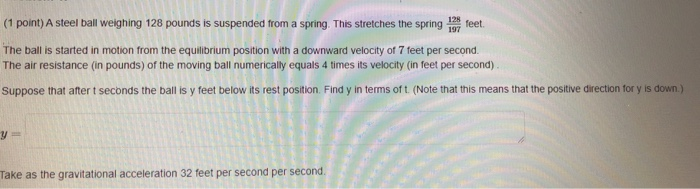Defined that natural length is unknown, can we assume its zero? do we need natural length? 128 feet 197 (1 point) A steel ball weighing 128 pounds is suspended from a spring. This stretches the spring The ball is started in motion from the equilibrium position with a downward velocity of 7 feet per second. The air resistance (in pounds) of the moving ball numerically equals 4 times its velocity (in feet per second) Suppose that after seconds the ballis...

• ### Problem 1. (point) A steel ball weighing 128 pounds is suspended from a spring. This stretches...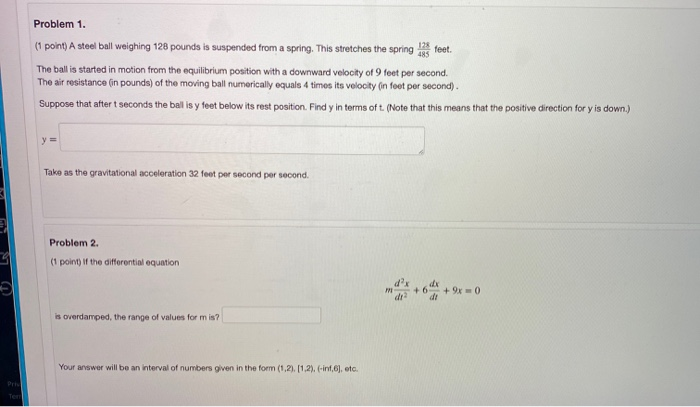Problem 1. (point) A steel ball weighing 128 pounds is suspended from a spring. This stretches the spring feet. The ball is started in motion from the equilibrium position with a downward velocity of 9 feet per second. The air resistance (in pounds) of the moving ball numerically equals 4 times its velocity on feet per second). Suppose that after t seconds the ball is y feet below its rest position. Find y in terms of t. (Note that this...

Free Homework App